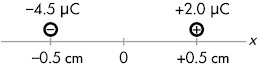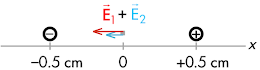## 20160316

### Physics quiz question: total electric field direction between two charges

Physics 205B Quiz 3, spring semester 2016
Cuesta College, San Luis Obispo, CATwo point charges are held at fixed positions. A –4.5 µC charge is at x = –0.5 cm, and a second +2.0 µC charge is at x = +0.5 cm. At x = 0, the electric field is directed to the:
(A) left.
(B) right.
(C) (There is no direction, as the electric field would be zero.)
(D) (Not enough information is given.)

Correct answer (highlight to unhide): (A)The electric field vector at x = 0 due to the –4.5 µC source charge is shown at right, where it points in towards this negative source charge.The electric field vector at x = 0 due to the +2.0 µC source charge is shown at right, where it points out away from this positive source charge. (The magnitudes of these electric field vectors are not quite to scale.)The total electric field at x = 0 due to both source charges is the vector addition of the individual electric field vectors at that location. Since the electric fields due to the –4.5 µC source charge and the +2.0 µC source charge both point in the same direction (to the left) at x = 0, the direction of the total electric field there would also point to the left, and the magnitude of the total electric field there would be the sum of the two individual electric field magnitudes.

Sections 30882, 30883
Exam code: quiz03Ccf7
(A) : 23 students
(B) : 10 students
(C) : 7 students
(D) : 0 students

Success level: 58%
Discrimination index (Aubrecht & Aubrecht, 1983): 0.48